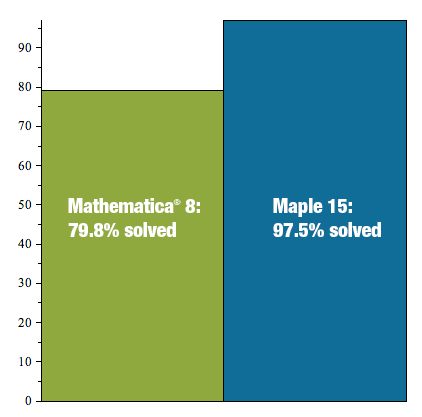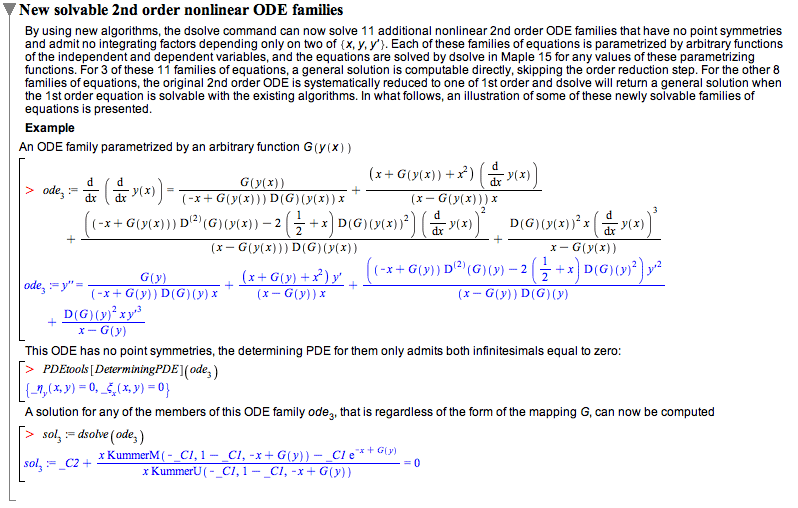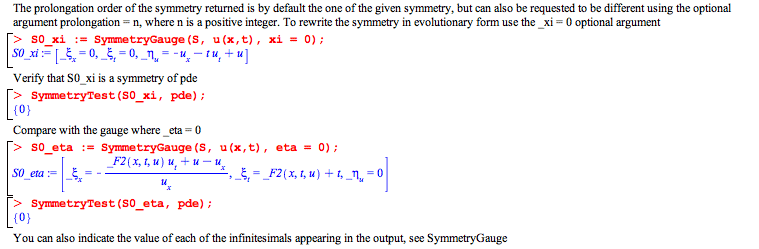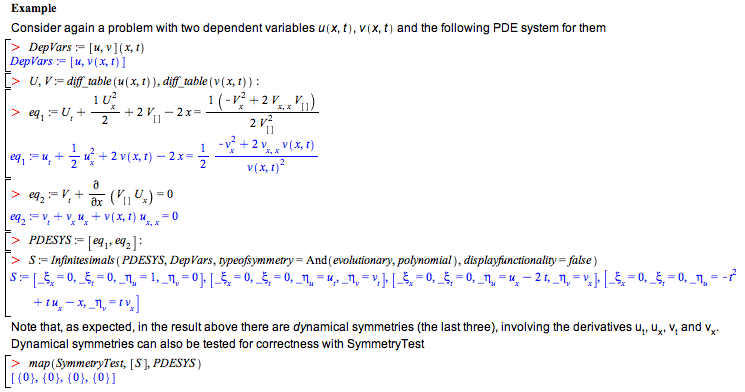New Features in Maple 15: Differential EquationsMaple is the uncontested leader for computing symbolic solutions to differential equations. Numerous improvements in Maple 15 further expand the classes of problems that can be handled.

Kamke Benchmark
Ordinary Differential Equations
Partial Differential Equations
PDE ToolsKamke Benchmark
Maple 15 now computes symbolic solutions to 97.5% of the 1345 solvable linear and nonlinear ODEs from the famous text, Differentialgleichungen by Kamke. Mathematica® 8 only handles 79.8%. Maple also solves these ODEs almost 10 times faster than Mathematica.Looking at the details, Maple is the only system to solve any of the systems in some classes of ODEs:Kamke (1345 solvable ODEs) Mathematica 8 Maple 15 1st order ODEs (576) 86% (solved in 234 minutes) 99% (solved in 26 minutes) 2nd order linear ODEs (448) 89.7% (39min) 99.5% (2.3min) 2nd order nonlinear ODEs (188) 70% (78min) 97% (14min) 2nd order reducible nonlinear ODEs (58) 0% (23min) 86% (3min) 3rd order linear ODEs (85) 70% (23min) 91% (2.5min) 4th order linear ODEs (45) 77% (5.8min) 93% (0.5min) 5th order linear ODEs (12) 75% (7.4min) 92% (0.5min) High-order nonlinear ODEs (12) 64% (1.5min) 100% (0.6min) High-order reducible nonlinear ODEs (5) 0% (0.5min) 100% (0.2min) Total 79.8% (408min) 97.5% (49min)Ordinary Differential Equations (ODEs)
By using new algorithms, the dsolve command can now solve two additional 1st order and eleven 2nd order nonlinear ODE families, all of them parametrized by arbitrary functions of the independent and dependent variables and out of reach of the previously existing solving methods.Partial Differential Equations (PDEs)
A significant number of improvements and additions were made to the PDEtools package, setting a new benchmark for the state-of-the-art in symbolic computation and partial differential equation solving and symmetry analysis.

New commands: FunctionFieldSolutions, SymmetryCommutator and SymmetryGauge

Three new commands were added to PDEtools.

• FunctionFieldSolutions is a new command that involves an innovative approach to computing exact solutions to DE systems involving mathematical functions, possibly inequations, ODEs and also non-differential equations. In these cases, due to the presence of nonpolynomial objects, the original Maple approach was to decouple the system using differential polynomial extensions. This approach, although powerful, sometimes failed to solve the problem. The new approach in FunctionFieldSolutions skips that step entirely and instead searches for solutions that can be written as a power series (with some upper bound degree n) in the mathematical functions and its derivatives (up to some upper bound differential order m), having for coefficients multivariable polynomials (with some upper bound degree r). In turn these polynomials have undetermined coefficients that get adjusted, resulting in the solution. To compute the key values of n, m, r to construct these function field solutions, the new command maps the problem into one that can be tackled with the existing PDEtools:-Library:-UpperBounds (see PDEtools[Library]).
• SymmetryCommutator is a new command to compute the commutator between two symmetries, given either as lists of infinitesimals or as infinitesimal generator procedures. This command is useful when studying the properties of a group of symmetries or when deriving the group constants or relations to make the group complete.
• SymmetryGauge is a new command to gauge PDE symmetries. It is well known that an ODE symmetry can be rewritten in different ways (see Xgauge). It is not so well known, but the same happens with PDE symmetries. The ability to rewrite a symmetry is relevant for a number of purposes. First, that permits identifying that two apparently different symmetries are actually the same by rewriting them in evolutionary form (gauge ξ = 0). Second, symmetries that appear as dynamical ones can frequently be rewritten as pointlike, transforming the usability of the symmetry from perhaps very difficult into straightforward. Finally, depending on the form of the symmetry, by rewriting them it is frequently possible to simplify its form considerably so that invariants of canonical coordinates become computable and so the symmetry can be used to reduce the number of independent variables of PDE systems.New functionality in the PDEtools package
• The following commands were significantly enhanced in their functionality: ConservedCurrents, D_Dx, DeterminingPDE, Eta_k, InfinitesimalGenerator, Infinitesimals, IntegratingFactors, InvariantEquation and Solve.
• In Maple 15, the entire set of symmetry commands of PDEtools:
• Automatically use textbook mathematical jet notation on input and output;
• Handle list of infinitesimals or the corresponding symmetry generator operators in equal footing;
• Understand different jet notations as representing the same mathematical objects;
• Return list of infinitesimals with infinitesimal labels in mathematical notation, unless your input contains infinitesimals without labels;
• Return results in the jet notation of the input you pass to them;
• Optionally return in any jet notation you request.ExamplesNote the (new) presence of infinitesimal labels in this output. The motivation behind all these changes is to have a computational experience with symmetry analysis using true mathematical notation, as close as possible as the one you use when computing with paper and pencil.

Apart from the ability to compute conserved currents and integrating factors of general types, ConservedCurrents and IntegratingFactors can now specifically compute conserved currents and integrating factors of polynomial or functionfield types.Eta_k: the returned table procedure eta that provides any desired prolongation of the infinitesimals can now be indexed using independent and dependent variables in jet notation, or their respective numeric positions in the lists of independent and dependent variables (that is jetnumbers notation). Correspondingly, the output will be in jetvariables or jetnumbers notation. This implements a more natural mathematical representation of infinitesimal prolongations while providing an easy way to switch between notations, interactively or within a program,Infinitesimals understands more types of symmetries: evolutionary and general, apart from pointlike and contact, and can compute infinitesimals combining these types, as for instance in And(evolutionary, polynomial), that is, dynamical symmetries with the _xi infinitesimals equal to zero and the _eta infinitesimals polynomial in the jet variables including all partial derivativesInvariantEquation accepts a new optional argument, explicit, to indicate that the invariant equation to be returned should have the form of an explicit PDE system with all the highest derivatives isolated in the left-hand sides. In addition, this command can now compute the PDE system invariant under a set of generic dynamical symmetries, that is, symmetries where the corresponding infinitesimals depend directly on the derivatives of the unknowns of the problem.If you have Maple 15, you can try the examples on this page yourself: